Students can Download Maths Chapter 4 Information Processing Ex 4.4 Questions and Answers, Notes Pdf, Samacheer Kalvi 8th Maths Book Solutions Guide Pdf helps you to revise the complete Tamilnadu State Board New Syllabus and score more marks in your examinations.

## Tamilnadu Samacheer Kalvi 8th Maths Solutions Term 2 Chapter 4 Information Processing Ex 4.4

Information Processing in Maths 8th Standard  Miscellaneous Practice Problems

Information Processing Solution Question 1.
The rule of Fibonacci Sequence is F(n) = F(n – 2) + F(n – 1). Find the 11th to 20th Fibonacci numbers.
Solution:
Fibonacci series is given by F(n) = F(n – 2) + F(n – 1)
∴ F(11) = F(11 – 2) + F(11 – 1) = F(9) + F(10)
Similarly F(12) ; = F(12 – 2) + F(12 – 1) = F(11) + F(10)
F(13) = F(12) + F(11)
F(14) = F(13) + F(12)……
F(20) = F(19) + F(18)
The Fibonacci series given by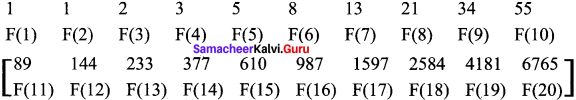8th Maths Book Information Processing Question 2.
In a library, 385 Math books, 297 Science books and 143 Tamil books are bundled equally in numbers. What is the maximum numbers of books possible in a bundle, for all types of books? (Use repeated division method)
Solution:
In library, no. of math books – 385
Science books – 297 .
Tamil books – 143
We need to find HCF by repeated division method.
Step 1:
First let us take the bigger 2 numbers which are 385 & 297 & find their HCF using repeated division method.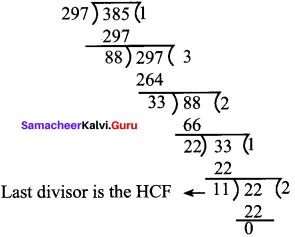Step 2:
Now let us find the HCF of 11 & 143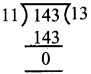∴ ’11’ is the HCF of 11 & 143
∴ Therefore, the HCF of 385, 297 & 143 is 11.
So, the maximum number of books possible in a bundle, for all types of books is 11.Repeated Subtraction Method Question 3.
Find the length of the largest which piece of wood used to measure exactly the lengths 4 m, 50 cm and 6 m 30 cm woods.( Use repeated subtraction method)
Solution:
Piece 1 of wood length = 4 m 50 cm ; 1m = 100 cm
∴ 4m 50 cm = 4 x 100 + 50 = 450 cm
Piece 2 of wood length = 6 m 30 cm = 6 x 100 + 30 = 630 cm
Now, we need to find the HCF of 450 & 630 by repeated subtraction method.
Let bigger number be m & smaller number be n
Therefore, m = 630 & n = 450
m – n = 630 – 450 = 180; now m = 450, n = 180
m – n = 450 – 180 = 270; now m = 180, n = 270
n – m = 270 – 180 = 90; now m = 180, n = 90
m – n = 180 – 90 = 90
∴ now m = n …90 is HCF
length of larget peice of wood to measure exactly the lenth of both pieces of wood is 90 cm

Question 4.
Using both repeated division method and repeated subtraction method and find the greatest number that divides 167 and 95, leaving 5 as reminder.
We need to find a number ‘x’ that divides 167 & 95 having 5 as reminder.
Therefore, if we subtract 5 from both the numbers, there wont be any reminder when the numbers are divided by ‘x’.
∴ x is the HCF of (167 – 5) & (95 – 5)
= 162 & 90
Finding HCF of 162 & 90 by repeated division method,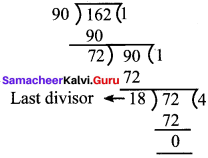∴ HCF of 162 & 90 is 18.
HCF of 162 & 90 using repeated subtraction method
Let m = 162 & n = 90
m – n = 162 – 90 = 72
m – n = 90 – 72= 18
m – n = 72 – 18 = 54
n – m = 54 – 18 = 36
n – m = 36 – 18 = 18
now m = n = 18
The number is 18
∴ 18 is the HCFQuestion 5.
Praveen recently got the registration number for his new two-wheeler. Here, the number is given in the form of mirror – image. Encode the image and find the correct registration number of praveen’s two – wheeler.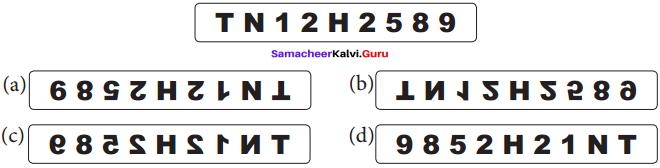Solution:
The mirror image is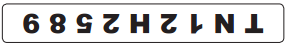When we place an imaginary mirror & visualize the image seen in the mirror, we will get the below.∴ The answer is Option c